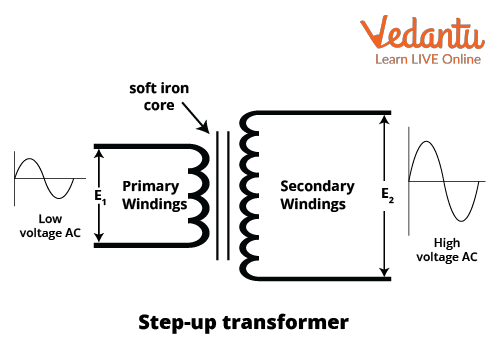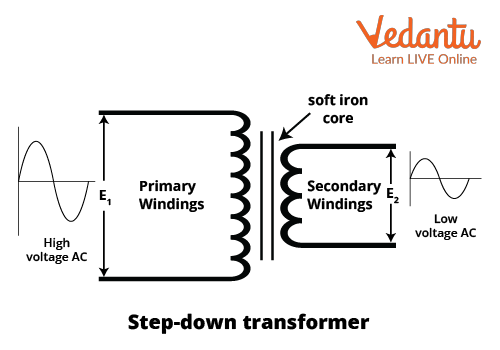Courses
Courses for Kids
Free study material
Free LIVE classes
More

# Step Up and Step Down Transformer for JEE## Introduction to Transformers

Last updated date: 27th Mar 2023
Total views: 84k
Views today: 0.60k

A Transformer, as the name implies, is a device used to transform something. So, a transformer is a passive component that transfers electrical energy among AC circuits. There is a part called the core in a transformer in which any fluctuating current gives rise to magnetic flux, thereby inducing an electromotive force around the core.

More importantly, the transformers are widely used to change the levels of AC voltage and for this application, they are categorized into two types that are namely step up and step down transformers. Sometimes, there are power losses along many power distribution lines due to fluctuation in wire resistances. To prevent such problems, we put forth a transformer that can control the output voltage thereby overcoming the fluctuations. This is why step up and step-down transformers are used. Let us dive deeper into it.

## What is a Step Up and Step Down Transformer?

### Step-Up Transformer:

A step-up transformer is a device which makes long-distance transmission of electrical energy economically efficient; it converts low voltage AC into high voltage AC, which decreases the loss of energy during transmission. In the process of voltage conversion, the output current is decreased so that the input and output power of the system would be equal.

Step-up transformers are mostly used in electrical equipment to create a balance between the low input voltage and required high voltage such as microwave ovens, inverters, stabilizers, and so on.

### Step-Down Transformer:

The type of transformer having output voltage less than the input voltage is called a step-down transformer. It converts high voltage AC into low voltage AC, keeping the input and output power the same. High voltage AC running in the transmission lines is converted into the Low Voltage AC by step down transformer for the successful operation of our home appliances.

For example, the doorbell operates at 16V, while our power circuit carries 230V – 110V, so this balance between input and output voltage is managed by a step-down transformer; it reduces the voltage from 220V to 16V.

Now, let us know about the construction process, working, and the difference between step and step down transformers.

## Construction of Step Up and Step Down Transformers

The major construction revolves around the two parts, the core and the windings.

Core: A transformer consists of a soft iron core and two inductive windings. The core is made up of soft iron with a collection of laminated metal sheets to facilitate the windings; laminated metal sheets are used to reduce the eddy current loss.

Windings: It has two windings primary and secondary winding; primary winding is connected to the input and secondary winding is connected to the output. Proper insulation is provided between both the coils and between the coil and core.  Here is the image of the primary and secondary winding of a step-up transformer.As shown in the figure, in a step-up transformer, the number of turns in secondary windings is greater than in primary windings, therefore it steps up the voltage, i.e., converts a primary voltage into a high secondary voltage.

Meanwhile, in step down transformers, the number of turns in the primary winding is greater than in the secondary winding. Therefore, it converts the voltage in a primary coil into a low secondary voltage. This is shown in the Image 2 below.Therefore, the step up and step down transformer diagram is discussed and now let us have a look at how to explain step up and step down transformers working.

## How Do Step Up and Step Down Transformers Work?

Step up and step-down transformers work on the principle of mutual induction. According to this, the changing current in one coil induces EMF in the other coil. Alternating current flows in one direction, stops reversing its direction, and then again flows in another direction. Alternating current flowing in the primary coil will create a varying magnetic field in the core of the transformer that in turn produces the alternating current in the secondary coil. Depending on the number of turns in the primary and secondary coil, the voltage is either stepped up or down.

In the step-down transformer, the number of turns is greater in the primary coil than in the secondary coil, so the output value will be lesser than the input and it converts high voltage AC into low voltage AC. In the step-up transformer, the number of turns in the secondary coil is greater than the primary coil and it converts low voltage AC into high voltage AC.

The number of turns in coils, current and voltage can be related by the equation below

$\dfrac{V_{s}}{V_{p}}=\dfrac{N_{s}}{N_{p}}=\dfrac{I_{p}}{I_{s}}$

Where $V_s$ and $V_p$ are the voltages of the secondary and the primary winding of the transformer respectively. $N_s$ and $N_p$ are the numbers of turns of the secondary and the primary windings. $I_s$ and $I_p$ are the currents induced in the windings.

Step up and step down transformers neither create nor destroy the electrical power. The input and output power remain the same by changing the voltage and current at the primary and secondary coils.

## Difference Between Step Up and Step Down Transformers

The below-given table can be used to distinguish between step up and step down transformers.

 Criteria Step Up Transformer Step Down Transformer Operation Increases voltage at the output. Decreases voltage at the output. Construction The number of turns in the secondary coil is greater than in the primary coil. The number of turns in the primary coil is greater than in the secondary coil. Voltage Low input voltage and high output voltage High input voltage and low output voltage Current High input current and low output current Low input current and high output current

There are applications of step-up and step-down transformers which we can witness in our daily life.

A Step-up transformer would be applicable in power transmission, microwave ovens, inverters, and so forth. Whereas a step-down transformer is used in power distribution, electrical devices such as bells, mobile chargers etc.

Let us discuss why there is a need for these transformers in our lives with an explicit explanation.

## Why Do We Need Step Up and Step-Down Transformers?

Electric poles outside our homes have a voltage of 11kv, but we get a supply to our home of 220 volts, which is then distributed to various electrical appliances. Have you ever wondered how this is possible? A voltage control device, called the transformer, is used to distribute the electrical energy by increasing or decreasing the voltage. Depending upon its operation, a transformer is divided into two types, a step up and step-down transformer. A step-up transformer is used in power transmission to convert low voltage AC into high voltage AC, to prevent power loss during transmission. On the other hand, a step-down transformer is used to convert high voltage AC into low voltage AC for the successful operation of low voltage devices.

## Conclusion

Step up and step-down transformers are used to create a balance between the supply and required voltage in electrical appliances. Step-up transformers are mainly used to reduce the loss of electrical energy during transmission, while step-down transformers are used to distribute the high supply voltage to the devices using low voltage for their operation. Step up and step-down transformers do not create or destroy the electrical energy, but only convert it. Therefore, we discussed things like what is a step up and step down transformer, the step up and step down transformer diagram and how to distinguish between a step up and step down transformer.

## FAQs on Step Up and Step Down Transformer for JEE

1. What is the principle of working for a step up and step down transformer?

Step up and step-down transformers work on the principle of mutual induction. Transformers are electrical devices which consist of primary and secondary windings on a laminated soft iron core; these windings are called primary and secondary coils. In transformers current flowing in the primary coil generates magnetic flux and this magnetic flux causes induction of EMF i.e. Electromotive force in the secondary coil of the transformer. Therefore, an induced voltage transforms from a primary winding to a secondary winding in the transformer.

2. What is the weightage of this topic for the JEE exam?

Step up and step-down transformer is an important topic from point of view, each year 2-3 are asked about the topic, numerical questions based on the efficiency of transformers are asked in JEE and you can also expect MCQs based on the working and application of transformers. So, step up and step transformers hold about 2-3% weightage for JEE exams. This topic is also important for the preparation for and other board exams as questions based on working and construction are also asked in previous year’s papers.

3. Can a step-up transformer be used as a step-down transformer? If yes, how?

Yes, a step-up transformer can be used as a step-down transformer but certain conditions for the connection of input and output should be followed which is by reversing the input and output connection. Normally in a step-up transformer input is connected to the primary coil of the transformer and output is connected with the secondary coil, to use a step-up transformer as a step-down transformer input should be connected with the secondary coil and output should be connected with the primary coil of the transformer.